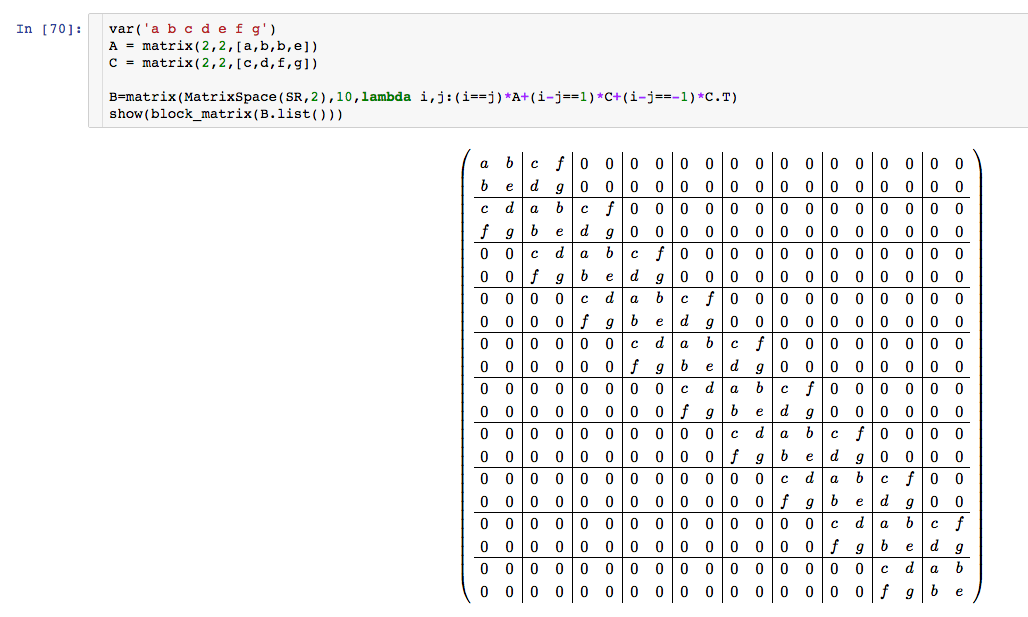# Create a band matrix with repeating and alternating values

I have 7 values: a, b, c, d, e, f and g

I would like to construct an m by n matrix in this way:

[ a b c d 0 0 0 0 . . . .]
[ b e f g 0 0 0 0 . . . .]
[ c f a b c d 0 0 . . . .]
[ d g b e f g 0 0 . . . .]
[ 0 0 c f a b c d 0 0 . .]
[ 0 0 d g b e f g 0 0 . .]
[ . . 0 0 c f a b c d . .]
[ . . 0 0 d g b e f g . .]


And so forth...

Therefore, the desired matrix is symmetrical. Values a and e alternate on the main diagonal; values b and f alternate on the 1st upper diagonal; values c and g alternate on the 2nd upper diagonal; values d and 0 alternate on the 3rd upper diagonal. I would like to be able to specify the matrix size with m by n parameters (even though the resulting matrix is non-square).

What would be an efficient way to construct this matrix?

edit retag close merge delete

Sort by » oldest newest most votedvar('a b c d e f g')
A = matrix(2,2,[a,b,b,e])
C = matrix(2,2,[c,d,f,g])
B = block_matrix(2,2, [A,C,C.transpose(),A])
M = matrix(SR,10,10)
for k in range(4):
M.set_block(2*k,2*k,B)
M

moremore